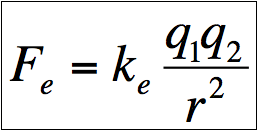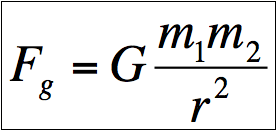## 10/27/09

Not really a clash of the titans so much as it is a way looking at the world as a whole.

The infinite scales that are looked upon in this world have such varying properties that depending on the scale being looked at can cause other scales' properties to become relatively meaningless. However, as physicists are continually search for the elusive theory of everything, theories at all scales become meaningful.

In an effort to showcase this, I took an example that most people have at least hopefully have heard of.

In comparing gravitational force with electrostatic force, I turn to mathematics in the comparison of the two forces between two protons.

The equation below is called Coulomb's law. It is used to calculate the electrostatic force.The equation below is called Newton's universal law of gravitation. It is used to calculate the gravitational force.The following information is pertinent in solving the force ratio.

proton mass = 1.67262E-27 kg
proton charge = 1.60218E-19 C
gravitational constant, G = 6.674E-11 m^3 kg^-1 s^-2
Coulomb's constant, k = 8.988E9 N m^2 C^-2

Looking at both equations, one can see that each has an r squared in the denominator. When comparing the ratios of the forces to each other, one can see that the electrostatic force is quite a bit orders of magnitude higher that gravity.This means that electrostatic force of two protons is 1,000,000,000,000,000,000,000,000,000,000,000,000 times greater than the gravitational force of two protons.

This makes it seem like gravity is almost meaningless in comparison. Yet, this begs the question, why is it that gravity is what holds us to the planet, planet in orbit around the sun as well as the moon around us along with the attraction of everything else is the universe?

At the atomic scale, electric charges of subatomic particles create such a powerful force due to its small distances. With opposite charges attracting with so much force, objects become inherently neutral. If objects are neutral in relation to each other, no other forces (outside of nuclear forces) will be acting upon them.

This causes gravity to win out. It may have lost the battle, but definitely not war. Gravity is always attractive whereas electrostatic force is attractive only if the charges are opposite of each other. That exception is what causes gravity to win out in large scales.

As the scale becomes smaller and smaller, electrostatic force becomes to take over. This along with the fact that opposing charges will coalesce creating larger and larger masses while becoming neutral. This leads to another reason why gravity will win the metaphorical war. The greater the masses become between the two objects, the greater the force of gravity becomes.

The varying degrees of forces attributable to each scale leads to varying areas of science, for example, cosmology and quantum mechanics.

Some people are just better at seeing the big picture...

while others like to think small.

Until next time (which is if and when I ever get a free moment).
Designed By Blogger Templates | Templatelib & Distributed By Blogspot Templates